Anzeige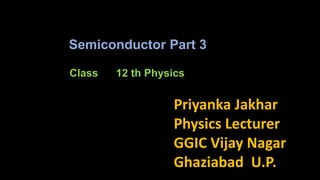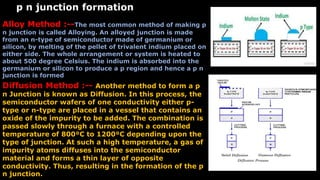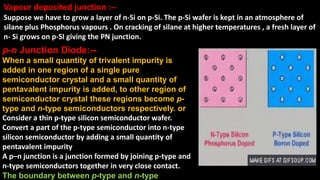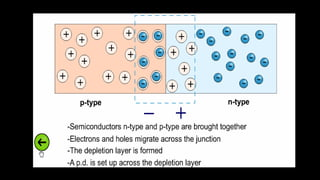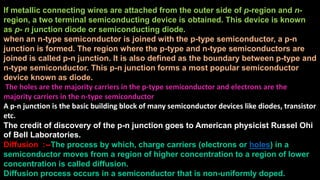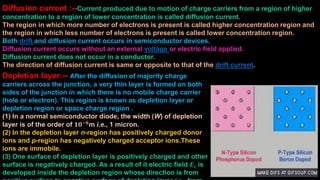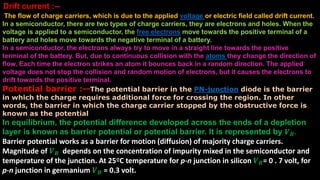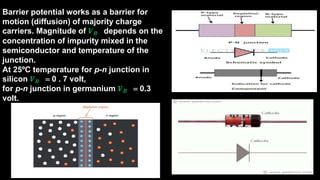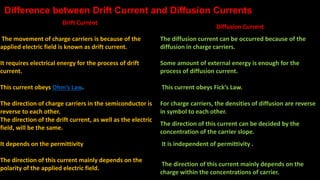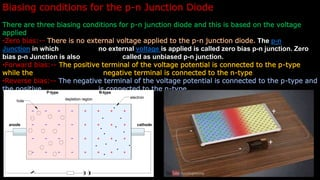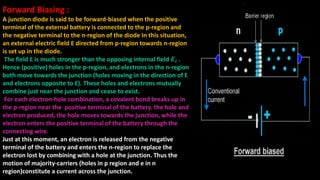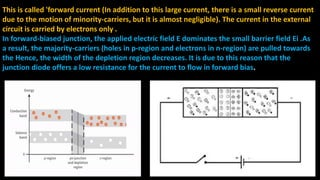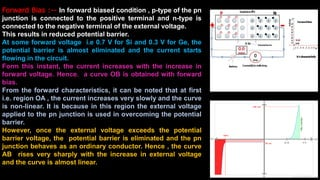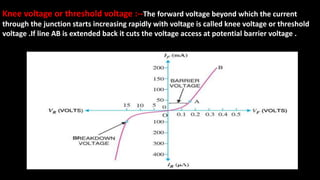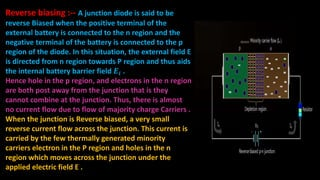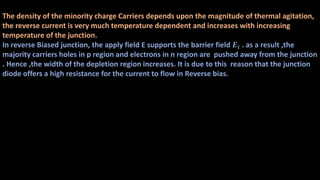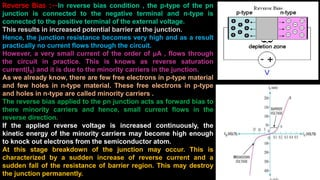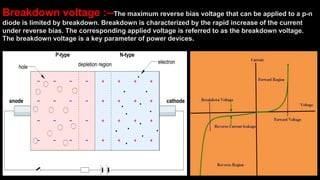1 von 18
Anzeige

### Class 12 th semiconductor part 3

1. Class 12 th Physics Priyanka Jakhar Physics Lecturer GGIC Vijay Nagar Ghaziabad U.P. Semiconductor Part 3
2. Alloy Method :--The most common method of making p n junction is called Alloying. An alloyed junction is made from an n-type of semiconductor made of germanium or silicon, by melting of the pellet of trivalent indium placed on either side. The whole arrangement or system is heated to about 500 degree Celsius. The indium is absorbed into the germanium or silicon to produce a p region and hence a p n junction is formed Diffusion Method :-- Another method to form a p n Junction is known as Diffusion. In this process, the semiconductor wafers of one conductivity either p- type or n-type are placed in a vessel that contains an oxide of the impurity to be added. The combination is passed slowly through a furnace with a controlled temperature of 800⁰C to 1200⁰C depending upon the type of junction. At such a high temperature, a gas of impurity atoms diffuses into the semiconductor material and forms a thin layer of opposite conductivity. Thus, resulting in the formation of the p n junction. p n junction formation
3. Vapour deposited junction :-- Suppose we have to grow a layer of n-Si on p-Si. The p-Si wafer is kept in an atmosphere of silane plus Phosphorus vapours . On cracking of silane at higher temperatures , a fresh layer of n- Si grows on p-SI giving the PN junction. p-n Junction Diode:-- When a small quantity of trivalent impurity is added in one region of a single pure semiconductor crystal and a small quantity of pentavalent impurity is added, to other region of semiconductor crystal these regions become p- type and n-type semiconductors respectively. or Consider a thin p-type silicon semiconductor wafer. Convert a part of the p-type semiconductor into n-type silicon semiconductor by adding a small quantity of pentavalent impurity A p–n junction is a junction formed by joining p-type and n-type semiconductors together in very close contact. The boundary between p-type and n-type
4. If metallic connecting wires are attached from the outer side of p-region and n- region, a two terminal semiconducting device is obtained. This device is known as p- n junction diode or semiconducting diode. when an n-type semiconductor is joined with the p-type semiconductor, a p-n junction is formed. The region where the p-type and n-type semiconductors are joined is called p-n junction. It is also defined as the boundary between p-type and n-type semiconductor. This p-n junction forms a most popular semiconductor device known as diode. The holes are the majority carriers in the p-type semiconductor and electrons are the majority carriers in the n-type semiconductor A p-n junction is the basic building block of many semiconductor devices like diodes, transistor etc. The credit of discovery of the p-n junction goes to American physicist Russel Ohi of Bell Laboratories. Diffusion :--The process by which, charge carriers (electrons or holes) in a semiconductor moves from a region of higher concentration to a region of lower concentration is called diffusion. Diffusion process occurs in a semiconductor that is non-uniformly doped.
5. Diffusion current :--Current produced due to motion of charge carriers from a region of higher concentration to a region of lower concentration is called diffusion current. The region in which more number of electrons is present is called higher concentration region and the region in which less number of electrons is present is called lower concentration region. Both drift and diffusion current occurs in semiconductor devices. Diffusion current occurs without an external voltage or electric field applied. Diffusion current does not occur in a conductor. The direction of diffusion current is same or opposite to that of the drift current. Depletion layer:-- After the diffusion of majority charge carriers across the junction, a very thin layer is formed on both sides of the junction in which there is no mobile charge carrier (hole or electron). This region is known as depletion layer or depletion region or space charge region . (1) In a normal semiconductor diode, the width (W) of depletion layer is of the order of 𝟏𝟎−𝟔m i.e., 1 micron. (2) In the depletion layer n-region has positively charged donor ions and p-region has negatively charged acceptor ions.These ions are immobile. (3) One surface of depletion layer is positively charged and other surface is negatively charged. As a result of it electric field 𝑬𝒊 is developed inside the depletion region whose direction is from
6. The flow of charge carriers, which is due to the applied voltage or electric field called drift current. In a semiconductor, there are two types of charge carriers, they are electrons and holes. When the voltage is applied to a semiconductor, the free electrons move towards the positive terminal of a battery and holes move towards the negative terminal of a battery. In a semiconductor, the electrons always try to move in a straight line towards the positive terminal of the battery. But, due to continuous collision with the atoms they change the direction of flow. Each time the electron strikes an atom it bounces back in a random direction. The applied voltage does not stop the collision and random motion of electrons, but it causes the electrons to drift towards the positive terminal. Potential barrier :--The potential barrier in the PN-junction diode is the barrier in which the charge requires additional force for crossing the region. In other words, the barrier in which the charge carrier stopped by the obstructive force is known as the potential In equilibrium, the potential difference developed across the ends of a depletion layer is known as barrier potential or potential barrier. It is represented by 𝑽 𝑩. Barrier potential works as a barrier for motion (diffusion) of majority charge carriers. Magnitude of 𝑽 𝑩 depends on the concentration of impurity mixed in the semiconductor and temperature of the junction. At 25ºC temperature for p-n junction in silicon 𝑽 𝑩= 0 . 7 volt, for p-n junction in germanium 𝑽 𝑩 = 0.3 volt. barrier. Drift current :--
7. Barrier potential works as a barrier for motion (diffusion) of majority charge carriers. Magnitude of 𝑽 𝑩 depends on the concentration of impurity mixed in the semiconductor and temperature of the junction. At 25ºC temperature for p-n junction in silicon 𝑽 𝑩 = 0 . 7 volt, for p-n junction in germanium 𝑽 𝑩 = 0.3 volt.
8. Drift Current Diffusion Current The movement of charge carriers is because of the applied electric field is known as drift current. The diffusion current can be occurred because of the diffusion in charge carriers. It requires electrical energy for the process of drift current. Some amount of external energy is enough for the process of diffusion current. This current obeys Ohm’s Law. This current obeys Fick’s Law. The direction of charge carriers in the semiconductor is reverse to each other. For charge carriers, the densities of diffusion are reverse in symbol to each other. The direction of the drift current, as well as the electric field, will be the same. The direction of this current can be decided by the concentration of the carrier slope. It depends on the permittivity It is independent of permittivity . The direction of this current mainly depends on the polarity of the applied electric field. The direction of this current mainly depends on the charge within the concentrations of carrier. Difference between Drift Current and Diffusion Currents
9. Biasing conditions for the p-n Junction Diode There are three biasing conditions for p-n junction diode and this is based on the voltage applied •Zero bias:-- There is no external voltage applied to the p-n junction diode. The p-n Junction in which no external voltage is applied is called zero bias p-n junction. Zero bias p-n Junction is also called as unbiased p-n junction. •Forward bias:-- The positive terminal of the voltage potential is connected to the p-type while the negative terminal is connected to the n-type. •Reverse bias:-- The negative terminal of the voltage potential is connected to the p-type and the positive is connected to the n-type.
10. Forward Biasing : A junction diode is said to be forward-biased when the positive terminal of the external battery is connected to the p-region and the negative terminal to the n-region of the diode In this situation, an external electric field E directed from p-region towards n-region is set up in the diode. The field E is much stronger than the opposing internal field 𝑬𝒊 . Hence (positive) holes in the p-region, and electrons in the n-region both move towards the junction (holes moving in the direction of E and electrons opposite to E). These holes and electrons mutually combine just near the junction and cease to exist. For each electron-hole combination, a covalent bond breaks up in the p-region near the positive terminal of the battery. the hole and electron produced, the hole moves towards the junction, while the electron enters the positive terminal of the battery through the connecting wire. Just at this moment, an electron is released from the negative terminal of the battery and enters the n-region to replace the electron lost by combining with a hole at the junction. Thus the motion of majority-carriers (holes in p region and e in n region)constitute a current across the junction.
11. This is called 'forward current (In addition to this large current, there is a small reverse current due to the motion of minority-carriers, but it is almost negligible). The current in the external circuit is carried by electrons only . In forward-biased junction, the applied electric field E dominates the small barrier field Ei .As a result, the majority-carriers (holes in p-region and electrons in n-region) are pulled towards the Hence, the width of the depletion region decreases. It is due to this reason that the junction diode offers a low resistance for the current to flow in forward bias.
12. Forward Bias :-- In forward biased condition , p-type of the pn junction is connected to the positive terminal and n-type is connected to the negative terminal of the external voltage. This results in reduced potential barrier. At some forward voltage i.e 0.7 V for Si and 0.3 V for Ge, the potential barrier is almost eliminated and the current starts flowing in the circuit. Form this instant, the current increases with the increase in forward voltage. Hence. a curve OB is obtained with forward bias. From the forward characteristics, it can be noted that at first i.e. region OA , the current increases very slowly and the curve is non-linear. It is because in this region the external voltage applied to the pn junction is used in overcoming the potential barrier. However, once the external voltage exceeds the potential barrier voltage, the potential barrier is eliminated and the pn junction behaves as an ordinary conductor. Hence , the curve AB rises very sharply with the increase in external voltage and the curve is almost linear.
13. Knee voltage or threshold voltage :--The forward voltage beyond which the current through the junction starts increasing rapidly with voltage is called knee voltage or threshold voltage .If line AB is extended back it cuts the voltage access at potential barrier voltage .
14. Reverse biasing :-- A junction diode is said to be reverse Biased when the positive terminal of the external battery is connected to the n region and the negative terminal of the battery is connected to the p region of the diode. In this situation, the external field E is directed from n region towards P region and thus aids the internal battery barrier field 𝑬𝒊 . Hence hole in the p region, and electrons in the n region are both post away from the junction that is they cannot combine at the junction. Thus, there is almost no current flow due to flow of majority charge Carriers . When the junction is Reverse biased, a very small reverse current flow across the junction. This current is carried by the few thermally generated minority carriers electron in the P region and holes in the n region which moves across the junction under the applied electric field E .
15. The density of the minority charge Carriers depends upon the magnitude of thermal agitation, the reverse current is very much temperature dependent and increases with increasing temperature of the junction. In reverse Biased junction, the apply field E supports the barrier field 𝑬𝒊 . as a result ,the majority carriers holes in p region and electrons in n region are pushed away from the junction . Hence ,the width of the depletion region increases. It is due to this reason that the junction diode offers a high resistance for the current to flow in Reverse bias.
16. Reverse Bias :--In reverse bias condition , the p-type of the pn junction is connected to the negative terminal and n-type is connected to the positive terminal of the external voltage. This results in increased potential barrier at the junction. Hence, the junction resistance becomes very high and as a result practically no current flows through the circuit. However, a very small current of the order of μA , flows through the circuit in practice. This is knows as reverse saturation current(IS) and it is due to the minority carriers in the junction. As we already know, there are few free electrons in p-type material and few holes in n-type material. These free electrons in p-type and holes in n-type are called minority carriers . The reverse bias applied to the pn junction acts as forward bias to there minority carriers and hence, small current flows in the reverse direction. If the applied reverse voltage is increased continuously, the kinetic energy of the minority carriers may become high enough to knock out electrons from the semiconductor atom. At this stage breakdown of the junction may occur. This is characterized by a sudden increase of reverse current and a sudden fall of the resistance of barrier region. This may destroy the junction permanently.
17. Breakdown voltage :--The maximum reverse bias voltage that can be applied to a p-n diode is limited by breakdown. Breakdown is characterized by the rapid increase of the current under reverse bias. The corresponding applied voltage is referred to as the breakdown voltage. The breakdown voltage is a key parameter of power devices.
Anzeige